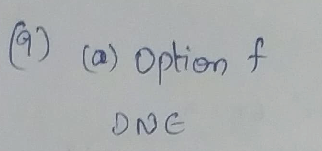Question

Calculus

The graph of a function f is shown.

Does f satisfy the hypotheses of the Mean Value Theorem on the interval [0, 5]?

a) Yes, because f is continuous on the open interval (0, 5) and differentiable on the closed interval [0,5]. b) Yes, because f is continuous on the closed interval [0, 5] and differentiable on the open interval (0, 5). c) Yes, because f is increasing on closed interval [0, 5]. d) No, because f does not have a minimum nor a maximum on the closed interval [0, 5]. e) No, because f is not differentiable on the open interval (0, 5). f) No, because f is not continuous on the open interval (0, 5).

If so, find a value c that satisfies the conclusion of the Mean Value Theorem on that interval. (If an answer does not exist,enter DNE.)Verified### Question 45629Calculus

III. The following Theorem was discussed in thesection about infinite series

### Question 45628Calculus

II. The following figure shows an infinite zigzag path with each zag occurs at an angle of t/4. Find the total length of this infinite zigzag path.

### Question 45627Calculus

I. Find the total area of the infinitely many triangles shown in the figure below.

### Question 45477Calculus

The bandwidth of a video being delivered on the internet has a continuous distributionon the interval (0,4), with a probability density function (PDF)
f_{X}(x)=\left\{\begin{array}{ll} \frac{c}{(1+x)^{2}} & \text { for } 0<x<4 \\ 0 & \text { otherwise. } \end{array}\right.
What is the probability that the bandwidth of a video is less than 0.6.

### Question 45476Calculus

You are given that P(A) = 0.5 and P(A U B) = 0.7. Student 1 assumes that A and B are independent and calculates P(B) based on that assumption. Student 2 assumes that A and B are mutually exclusive and calculates P(B) based on that assumption.Find the absolute difference between the two calculations.

### Question 45456Calculus

\text { Sketch a (relatively decent) contour diagram for } f(x, y)=\frac{y+1}{x-y} \text { which includes the }
c= 0, 1, 2, –2 level curves. (Pick a reasonable scale for your axes.)

### Question 45455Calculus

1. (3) Is there a function of two variables whose z = 0 level curve consists exactly of the circles x2 + y2 = 2 and x2 + y2= 9? If so, what is an example? If not, why not?

### Question 45454Calculus

\text { a) Consider the sphere } S:(x-2)^{2}+(y+3)^{2}+(z-1)^{2}=16
(b) Let P be the horizontal plane which passes through (4, 5, 2). Find the equation ofthe circle where P intersects S.
(a) Find anequation for the circle where S intersects the xz-plane.
(c) Find the point(s) where S intersects the x-axis.

### Question 45453Calculus

2. (5) Consider the (somewhat strange) function V (w) which returns the number of uniquevowels (a, e, i, o, u only) in the English word w.
(a) What is V(calculus)? V (brachiosaurus)?
(b) Describe (in words) the zeros of the function V, and giveexample.one
(c) What is the range of V?

### Question 45452Calculus

\text { Consider the function } f(x, y)=x-y^{2}+3 \text {. Sketch the cross-section of } f(x, y) \text { with }
\text { (a) } x=2
\text { (b) } y=-1

### Submit query